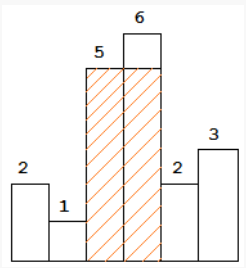# Painter's Partition Problem

Posted: 10 Jan, 2021
Difficulty: Moderate

## PROBLEM STATEMENT

#### You are supposed to return the area of the minimum time to get this job done of painting all the ‘N’ boards under a constraint that any painter will only paint the continuous sections of boards.

##### For example :
``````In the below figure where array/list elements are {2, 1, 5, 6, 2, 3}.
````````````A painter can paint blocks {5,6} or {1,5,6,2} together but not {2,5,6} or {5,6,3}.
``````
##### Input format :
``````The first line contains a single integer ‘T’ denoting the number of test cases.

The first line of each test case contains two integers ‘N’ and ‘K’ denoting the number of elements in the array/list and number of painters available.

The second line contains ‘N’ single space-separated integers denoting the elements of the array/list.
``````
##### Output format :
``````For each test case, print the minimum time required to get the job done.
``````
##### Note :
``````You do not need to print anything; it has already been taken care of.
``````
##### Constraints :
``````1 <= T <= 5
1 <= N <= 10^4
1 <= K <= N
1 <= ARR[i] <= 10^5

Where ‘T’ is the number of test cases.
'N' is the length of the given array/list (boards).
‘K’ is the number of painters available.
And, ARR[i] denotes the i-th element in the array/list.

Time Limit: 1 sec.
``````Approach 1

All we need to do is to Divide the boards into k of fewer partitions such that the maximum sum of the elements in a partition, overall partitions is minimized.

We can put the (k - 1)th divider between the ith and (i + 1)th element where i is between 1 and N.

Now the recursive function will just require two conditions that can lead to the result and that will be out the maximum total cost:

• The time is taken in the last partition where the (k - 1)th divider is before element i.
• The maximum cost of any partition already formed to the left of the (k - 1)th divider.

Now to avoid the exponential time complexity we can use Dynamic Programming since it already keeps a track of pre-calculated data and will lead to better results if we can take the data from the dp table.